Home » wave function

# wave function

« Back to Glossary Index

A wave function, in quantum mechanics, is an equation. It describes the behavior of quantum particles, usually electrons. Here “function” is used in the sense of an algebraic function, that is, a certain type of equation. A function is like a little machine that if you feed in a certain number, the machine will “massage” it in a specified way and output a certain number.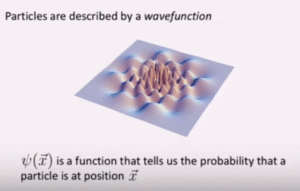[Image source: LMS Popular Lecture Series 2017, ‘The Unreasonable Effectiveness of Physics in Maths’, David TongLondon Mathematical Society Published on Oct 3, 2017 https://www.youtube.com/watch?v=UVuKyZ4pBzg&t=2068s]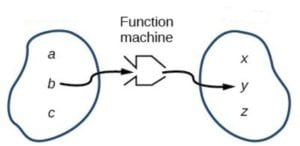A function is a kind of equation. It’s a little mathematical machine that converts certain numbers to other numbers by following a rule. (Image source: Jay Abramson, et al. Precalculus; via OpenStax at http://cnx.org/contents/fd53eae1-fa23-47c7-bb1b-972349835c3c@5.175 ; Creative Commons License)

It’s a wave function because it takes the form of equations that usually describe waves. Physicists can look at it and say, “I know what this equation is describing; it’s describing a wave.”

The symbol for the wave function in quantum mechanics is Ψ, the ancient Greek letter that is written  “psi” and pronounced “sigh.”

## The Quantum Wave Function and Schrodinger’s Equation

The wave function is an equation or a set of equations derived from Schrodinger’s Equation. Schrodinger’s Equation does not calculate the behavior of quantum particles directly. First it must be used to generate a wave function(s). It’s the wave function that actually describes the behavior of quantum particles.

## The Wave Function Calculates the Behavior of “Slow” Particles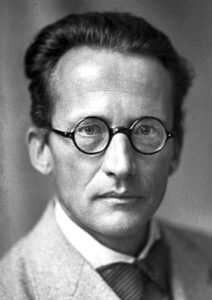Erwin Schrodinger in 1933, (1887-1961), author of the Schrodinger Equation. [Image source: The Nobel Foundation via https://commons.wikimedia.org/w/index.php?curid=6209244]The wave function can be used to calculate and predict the results of experiments conducted on subatomic particles. Usually, it is used only to describe the behavior of electrons because it is not accurate for faster-moving particles. Faster-moving particles, those moving at a few percentages of the speed of light or faster, are noticeably affected by Special Relativity. But the Schrodinger Equation does not take Special Relativity into account. Since the 1920’s, the Schrodinger’s Equation has been upgraded a couple of times to take into account Special Relativity as well as other factors. It now takes the form of Quantum Electrodynamics (QED), which can be used to calculate the behavior of both slow and faster-moving particles.

The Schrodinger Equation and the derived wave function(s) continue to be commonly used for calculations in technological applications when particles are not traveling at a significant fraction of the speed of light.

## Example of a Wave Function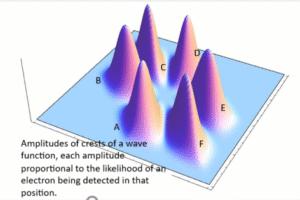Here’s an example of how the wave function works. Let’s say that we have an electron in a box, possibly imprisoned by magnetic fields. The wave function can be calculated which tells us the likelihood that the electron is in any particular position in the box. Let’s say that the magnetic fields are of equal strengths in six different positions within the box. We would feed into the wave function the strengths of the magnetic fields as well as other factors, do the calculations, and out would come the likelihood that we would find the electron in any particular position in the box. Note that the outcome of the calculation would be a set of probabilities as to the likely position in which we will detect the electron. Quantum mechanics is probabilistic.

Let’s say that the calculation of the wave function tells us that the electron has an equally high probability of being detected in six different positions and a negligible probability of being detected elsewhere. The accompanying image shows the graph of this result. It shows a three-dimensional wave with each “peak” representing a high probability of detecting the electron in that position.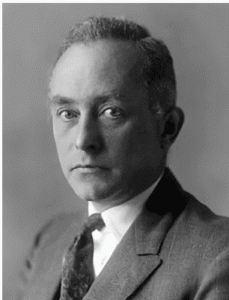Max Born (1882-1970), one of the founders of quantum mechanics and author of the Born Rule. [Image source: Public Domain, https://en.wikipedia.org/wiki/Max_Born]The height of each peak is called the “amplitude” of the wave in that position. The square of the amplitude gives the probability of detecting the electron in that position. This is the Born Rule, named after Max Born, who first published this rule of calculation in 1926.

Here is a highly schematic representation of the wave function imaged by this graph:

ψ =(1/6 Position A) + (1/6 Pos. B) + (1/6 Pos. C) + (1/6 Pos. D) + (1/6 Pos. E) + (1/6 Pos. F)

Here ψ symbolizes the wave function. It’s the result of summing the data for all six positions: Position A, Position B, Pos. C, etc. The wave amplitude for each position is the square root of 1/6 (written 1/6). According to the Born rule, we square the amplitude and get the probability that the electron will be detected in each position. In this case, for each position, it happens to be a probability of 1/6 or 16.7%. There is a 16.7% probability that the electron will be detected in Position A, a 16.7% probability that it will be detected in Position B, etc.

Of course, in other situations, the probabilities of particular outcomes might not be equal. For example, if the magnetic field were particularly strong in one corner of the box, it might push the electron away so as to reduce the likelihood of finding the electron in that corner.

The wave function can also be used to calculate many other properties of electrons, such as spin, energy, or momentum. For simplicity, this discussion focuses only on position.

## Physical Meaning of the Wave Function

A wave function is a piece of math, an equation. The physical meaning of the wave function is a matter of debate among quantum physicists. This debate includes the question of whether the wave function describes an actual physical wave.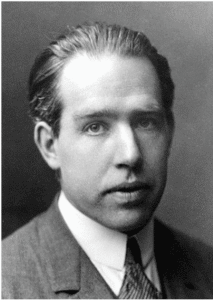Niels Bohr in about 1922, (1885-1962), Founding Father of quantum mechanics, developer of the Copenhagen Interpretation. [Image source: Public Domain, https://en.wikipedia.org/wiki/Niels_Bohr]The Copenhagen Interpretation, formulated in the 1920’s and ‘30’s, provides the original understanding of the wave function. According to this interpretation, the wave function is not describing a physical wave nor anything else that is physical. Several problems arise if one attempts to assign physicality to the wave function.*

In the Copenhagen Interpretation, the wave function describes a “probability wave.” But this is not a physical wave, it is some kind of mathematical item. How can a piece of math cause actions in the physical universe? The Copenhagen Interpretation does not answer this question. Instead it tells us not to ask it as it is not a “scientific” question. It is considered unscientific because it asks about something that we cannot observe: What is the quantum particle doing prior to detection? According to the philosophy from which the Copenhagen Interpretation grew, Logical Positivism, science should properly address only that which can be observed with scientific instruments.

Niels Bohr, godfather of quantum mechanics and of the Copenhagen Interpretation, explained that, “It is wrong to think that the task of physics is to find out how nature is. Physics concerns what we can say about nature….” Bohr wanted to discuss mathematics (“what we can say about nature”) not nature itself. He was concerned about mapping the territory in such a way that the map is useful for guiding travelers. He did not feel that science should concern itself with the issue of what the territory is “really” like. The Schrodinger Equation and its wave function provide such a map.

Many physicists continue to adhere to the Copenhagen Interpretation. It’s also called the “Orthodox Interpretation” or sometimes, all too bluntly, the “Shut up and calculate” interpretation. But, as noted below, physicists have developed a number of alternatives to the Copenhagen Interpretation which address the issue of the physical reality of the wave function.

## Collapse of the Wave Function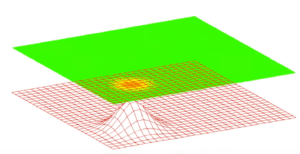The red mesh graphs the wave function of the electron. The green film represents, perhaps, a fluorescent screen. Upon detection by the fluorescent screen, the electron creates a tiny flash at a single point (yellow/orange dot). Wave function collapse is transformation from a wave to a dot (particle). [Image source: stills from Fermilab video by Dr. Don Lincoln, “Quantum Field Theory” (in the public domain) Jan. 14, 2016; https://www.youtube.com/watch?v=FBeALt3rxEA&feature=youtu.be.]Upon detection of the quantum particle, the probabilities calculated by the wave function instantaneously convert to a 100% probability for the position in which the electron is detected and 0% everywhere else. This is called the “collapse of the wave function.” Wave function collapse is the transformation from a spread-out wave described by the wave function to a localized particle. It is an aspect of the wave/particle duality of quantum mechanics.

The wave function can’t be used to calculate how the probabilities change upon particle detection. In the example above, if the electron were detected in Position A, the question arises: Where in the wave equation does it tell us to change the percentage from 16.7% for Position A to 100% ? Nowhere–nothing in the math tells us this. Physicists must “write in by hand” the detected position of the electron rather than being able to calculate it.

This is puzzling to physicists. They expect that if they fully understand a phenomenon, they should be able to calculate its behavior. In Newtonian physics, physicists are able to calculate exact positions of objects, given sufficient data. The inability to calculate aspects of the collapse of the wave function and why a measurement instrument should cause this collapse is called the “Measurement Problem.”

However, over many repetitions of the experiment, perhaps thousands, the wave function gives valid information. It tells us the percentages of electrons that will be found in specific positions. In the example given above, it tells us that 16.7% of the electrons will be detected at each of the six peaks of the wave function. So, many physicists have come to accept the use of the wave function without fully understanding the physical reality that it describes. This is the “Shut up and calculate” approach.

## The Wave Function as a Physical Thing

In contrast to the Copenhagen Interpretation, some of the other 15 or so interpretations of quantum mechanics assign physical reality to the wave function. The de Broglie-Bohmian Interpretation holds that the wave function describes a physical wave associated with the electron and that this wave involves a new type of energy. The electrons are like surfers on a wave that guides them to their destination. The wave creates the quantum wave-like effects, and the electrons create the particle effects. There is always a wave and always particles. No wave function collapse from wave to particle occurs. For more on this interpretation, see measurement in quantum mechanics.

The Many Worlds interpretation holds that the wave function describes the probabilities of events occurring in many different universes. And the Transactional Interpretation holds that the wave function describes a sublevel of reality in which quantum events occur. This is quite an assortment of interpretations to choose from, and, actually, there are many more!

In the following quote, Brian Greene, a physicist and science writer, summarizes the debate about the physical meaning of the wave function. He uses the Copenhagen Interpretation’s term for what the wave function is describing, a “probability wave,” and writes:

“Whether we should say that an electron’s probability wave is the electron, or that it’s associated with the electron, or that it’s a mathematical device for describing the electron’s motion, or that it’s the embodiment of what we can know about the electron is still debated.” (Italics per original)

## Problems with Physicality of the Wave Function

• The wave function can include the imaginary number i, that is the square root of negative 1. But no number multiplied by itself equals negative 1. So, what physical thing could can be described by the square root of negative 1? As Dr. Ruth E. Kastner writes, “Anything observable in the world of appearance must be described by real numbers [not imaginary numbers]….”
• We know of no medium in which the guiding wave is traveling. A wave is nothing more than a vibration. What is vibrating? Some people say that quantum waves are unique in that they don’t require a medium. But these words only paper over this logical problem.
• If an interaction of two or more particles is being described, the wave function includes more than three spatial dimensions. As current understanding of the physical universe includes only three spatial dimensions, the wave, as described, doesn’t seem to fit into physical reality.

These properties of the wave function lead to the conclusion that it is describing something other than a physical wave.

This site uses Akismet to reduce spam. Learn how your comment data is processed.